Next: 3.2 Material Models Up: 3.1 The Device Simulator Previous: 3.1.3 Hydrodynamic Model Current

## 3.1.4 Lattice Heat Flow Equation

Additionally the heat flux equation is solved self consistently with the DD or HD transport equations. The heat flux equation reads:

 div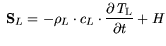(3.15)

and is solved in combination with Fourier's law: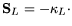grad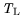(3.16)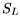denotes the lattice heat flux density,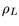the mass density,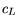the heat capacity, and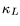the thermal conductivity.represents the lattice temperature. The implementation of the heat generation term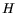depends on the transport model. For the DD case the carriers are assumed to energetically relax instantly which is equivalent to an infinitely small relaxation time. Thus, they deposit the energy accepted by the field instantly to the lattice. For the recombination heat it is assumed that every carrier provides the energy equivalent to the band gap energy . Thus, the generation termreads:grad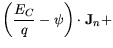grad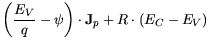(3.17)

For the hydrodynamic case forthe following term is used: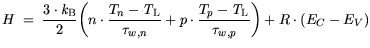(3.18)

(3.19) is obtained from the stationary energy flux balance derived from the second moment solving the Boltzmann equation and the carrier continuity equations (3.2) and (3.3) for the hydrodynamic model used [49,147]. The first two terms of (3.19) represent energy relaxation approaches approximating the scattering terms of the Boltzmann equation . The third term represents an effective recombination term. For the recombination a similar approximation is taken as in the DD case. This is sound and introduces a small error only, as the recombination energies generally are smaller than the Joule like contribution in (3.18) and (3.19). This is different for intensive light irradiation where additional contributions have to be added in order to model the effective recombination term.Next: 3.2 Material Models Up: 3.1 The Device Simulator Previous: 3.1.3 Hydrodynamic Model Current
Quay
2001-12-21